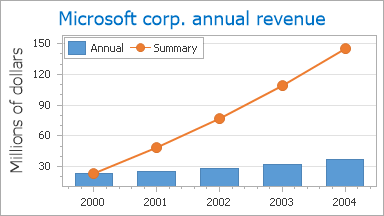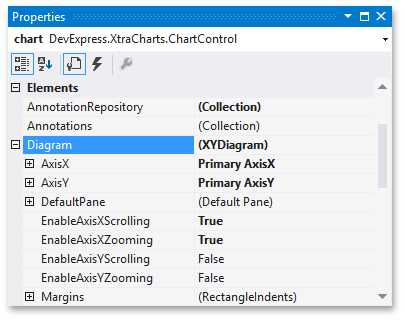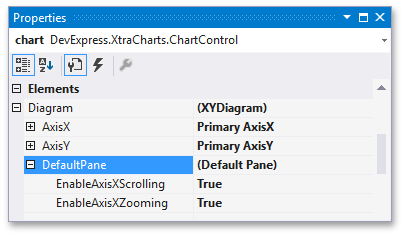20.1
19.2
19.1
18.2
18.1
17.2

# XY-Diagram

This document details the specifics of the XY-Diagram type. It lists the series view types associated with this diagram type, demonstrates how its specific options can be accessed (both at design and runtime), and briefly describes these options. Before reading this text, you may wish to review the basics of using diagram in the WinForms Chart Control.

This document consists of the following sections.

## Associated Series View Types

The XY-Diagram is the most common diagram type, since it's used to plot the series which are displayed using both axes: the axis of arguments (X-axis) and the axis of values (Y-axis). There are many such series view types available in XtraCharts. You can freely plot multiple series of these view types together, within the same diagram object, as shown in the following image.In particular, the XY-Diagram is used to plot series of the following view types:

## Accessing a Diagram

To access the XY-Diagram's options at design time, click your chart, to select it. Then, in the Properties window, expand the ChartControl.Diagram property.At runtime, cast your instance of the Diagram object to the XYDiagram type.

``````
// Cast Diagram to the XYDiagram type.
XYDiagram diagram = chart.Diagram as XYDiagram;
if (diagram != null) {
// Access diagram properties, for example rotate the diagram.
diagram.Rotated = true;
// Access properties of objects that belong to the diagram,
// for example axes and panes.
diagram.AxisY.Title.Text = "Population mid-year, millions";
diagram.DefaultPane.Weight = 2;
}
``````

## Specific Options

The available options, specific for the XY-Diagram type, are briefly described below. For the more detailed information about them, refer to these properties' descriptions.

In addition, it's possible to control the diagram's appearance. Since XY-Diagram supports panes, there is a difference on how the appearance properties of this diagram are accessed. So, for the default pane, they can be accessed via the XYDiagram2D.DefaultPane property, as shown in the following image.These properties are listed below. Note that these properties are also available for additional panes, the collection of which can be accessed via the XYDiagram2D.Panes property.